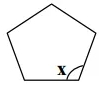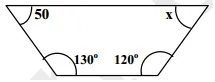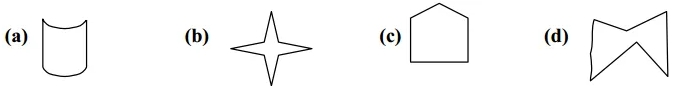# MCQ Questions for Class 8 Maths Chapter 4 Practical Geometry with Answers

Check the below NCERT MCQ Questions for Class 8 Maths Chapter 4 Practical Geometry with Answers Pdf free download. MCQ Questions for Class 8 Maths with Answers were prepared based on the latest exam pattern. We have provided Practical Geometry Class 8 Maths MCQs Questions with Answers to help students understand the concept very well. https://mcqquestions.guru/mcq-questions-for-class-8-maths-chapter-4/

Students can also refer to NCERT Solutions for Class 8 Maths Chapter 4 Practical Geometry for better exam preparation and score more marks.

## Practical Geometry Class 8 MCQs Questions with Answers

Practical Geometry Class 8 MCQ Question 1.
How many sides does decagon has?
(a) 8
(b) 10
(c) 6
(d) 12

Class 8 Maths Chapter 4 MCQ Question 2.
How many measurements can determine a quadrilateral uniquely?
(a) 2
(b) 3
(c) 4
(d) 5

MCQ Questions For Class 8 Maths Chapter 4 Question 3.
The diagonals of a square are ______________ each other
(a) equal to
(b) unequal to
(c) perpendicular bisectors of
(d) none of these

Class 8 Maths Ch 4 MCQ Question 4.
The opposite angles of a parallelogram are ______________ .
(a) Unequal
(b) equal
(c) complementary
(d) supplementary

Class 8 Math Chapter 4 MCQ Question 5.
What is the sum of the measures of angles of a convex quadrilaterals?
(a) 180°
(b) 90°
(c) 360°
(d) 45°

MCQ On Practical Geometry Class 8 Question 6.
How many diagonals does a regular Hexagon has ?
(a) 2
(b) 9
(c) 3
(d) 5

Maths Class 8 Chapter 4 MCQ Question 7.
How many sides does a heptagon have ?
(a) 2
(b) 4
(c) 7
(d) 5

Class 8 Maths Chapter 4 MCQ Online Test Question 8.
Minimum possible interior angle in a regular polygon is ______________.
(a) 70°
(b) 60°
(c) 90°
(d) 120°

Class 8 Chapter 4 Maths MCQ Question 9.
The diagonals of a square bisect each other at ______________ angle.
(a) acute
(b) right
(c) obtuse
(d) reflex

MCQ Class 8 Maths Chapter 4 Question 10.
the diagonals of a rhombus bisect each other at ______________ angles.
(a) acute
(b) right
(c) obtuse
(d) reflex

Ncert Class 8 Maths Chapter 4 MCQ Questions Question 11.
A parallelogram each of whose angles measures 90° is ______________.
(a) rectangle
(b) rhombus
(c) kite
(d) trapezium

Ncert Class 8 Maths Chapter 4 MCQ Question 12.
The value of x in the following figure is(a) 100°
(b) 90°
(c) 108°
(d) 120°

MCQ Of Practical Geometry Class 8 Question 13.
The value of (x) in the following figure is(a) 120°
(b) 80°
(c) 100°
(d) 60°

Class 8 Ch 4 Maths MCQ Question 14.
Which of the following polygons is convex polygon?Chapter 4 Maths Class 8 MCQ Question 15.
The number of sides in a regular polygon is 15 , then measure of each exterior angle is
(a) 24°
(b) 36°
(c) 20°
(d) 18°

Chapter 4 Class 8 Maths MCQ Question 16.
The angle sum of all interior angles of a convex polygon of sides 7 is
(a) 180°
(b) 540°
(c) 630°
(d) 900°

Question 17.
Maximum number of right angles in a right angled triangle are
(a) 2
(b) 1
(c) 3
(d) 0

Question 18.
All the angles of a regular polygon are of ______________.
(a) 90°
(b) 60°
(c) equal measure
(d) equal length

Question 19.
Polygons that have any portions of their diagonals in their exteriors are called
(a) Squares
(b) triangles
(c) convex
(d) concave

Question 20.
A polygon with minimum number of sides is
(a) Pentagon
(b) Square
(c) triangle
(d) angle

Question 21.
How many measurements are required to construct a quadrilateral, uniquely?
(a) Four
(b) Five
(c) Six
(d) Three

Question 22.
To construct a quadrilateral, we need to know two diagonals and _____ sides.
(a) One
(b) Two
(c) Three
(d) All four sides

Question 23.
If two diagonals and three sides are given, then:
(a) A quadrilateral cannot be constructed
(b) A quadrilateral can be constructed
(c) Insufficient information
(d) Any polygon can be constructed

Question 24.
If two diagonals are given, then we can construct a:
(a) Rhombus
(b) Rectangle
(c) Kite
(d) Parallelogram

Hint:
The two diagonals of a rhombus bisect each other at 90 degrees.

Question 25.
To construct a parallelogram we need to know:
(a) Length of its parallel sides
(b) Measure of interior angles
(c) Two adjacent sides and one angle
(d) Two adjacent sides and two angles